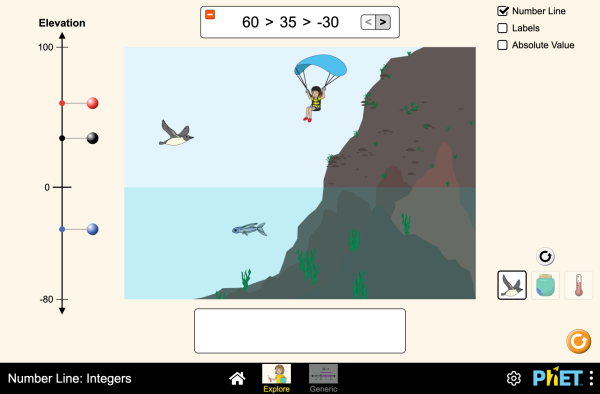# Number Line: Integers下载嵌入代码 关闭 嵌入模拟程序控制 你可以通过改变嵌入的代码改变程序的宽度和高度 插入一张图片，当点击这个图片时启动仿真程序。

### 主题

• 数轴
• Integers
• Absolute Value

### 描述

What do elevation, account balances, and temperature have in common? They can all be represented on a number line. Compare integers on a number line and in an inequality statement. Discover the meaning of absolute value.

### 示范 学习目标

• Use positive and negative numbers to represent quantities in multiple contexts.
• Explain the meaning of positive values, negative values, and zero, in multiple contexts.
• Describe the location of a point on a number line with respect to another number.
• Describe the location of a point on a number line with respect to its opposite
• Define the absolute value of a number as its distance from zero.
• Interpret inequality statements as statements about the relative position of two integers on a number line diagram.

### 标准对齐

#### Common Core - Math

6.NS.C.5
Understand that positive and negative numbers are used together to describe quantities having opposite directions or values (e.g., temperature above/below zero, elevation above/below sea level, credits/debits, positive/negative electric charge); use positive and negative numbers to represent quantities in real-world contexts, explaining the meaning of 0 in each situation.
6.NS.C.6a
Recognize opposite signs of numbers as indicating locations on opposite sides of 0 on the number line; recognize that the opposite of the opposite of a number is the number itself, e.g., -(-3) = 3, and that 0 is its own opposite.
6.NS.C.6c
Find and position integers and other rational numbers on a horizontal or vertical number line diagram; find and position pairs of integers and other rational numbers on a coordinate plane.
6.NS.C.7
Understand ordering and absolute value of rational numbers.
6.NS.C.7a
Interpret statements of inequality as statements about the relative position of two numbers on a number line diagram. For example, interpret -3 > -7 as a statement that -3 is located to the right of -7 on a number line oriented from left to right.
6.NS.C.7b
Write, interpret, and explain statements of order for rational numbers in real-world contexts. For example, write -3 oC > -7 oC to express the fact that -3 oC is warmer than -7 oC.
6.NS.C.7c
Understand the absolute value of a rational number as its distance from 0 on the number line; interpret absolute value as magnitude for a positive or negative quantity in a real-world situation. For example, for an account balance of -30 dollars, write |-30| = 30 to describe the size of the debt in dollars.
6.NS.C.7d
Distinguish comparisons of absolute value from statements about order. For example, recognize that an account balance less than -30 dollars represents a debt greater than 30 dollars.
7.NS.A.1a
Describe situations in which opposite quantities combine to make 0. For example, a hydrogen atom has 0 charge because its two constituents are oppositely charged.
7.NS.A.1b
Understand p + q as the number located a distance |q| from p, in the positive or negative direction depending on whether q is positive or negative. Show that a number and its opposite have a sum of 0 (are additive inverses). Interpret sums of rational numbers by describing real-world contexts.

### 教师指南仿真程序控制概述,简化模型,学生视角的思考 ( PDF ).

### 教师提交的活动

SECUNDARIA: Alineación PhET con programas de la SEP México (2011 y 2017)Diana López 高中

PRIMARIA: Alineación con programas de la SEP México (2011 y 2017)Diana López K-5

### HTML5 程序：

iOS 8+ Safari

Android:

Chromebook:

Windows 系统:

Macintosh 系统:
OS 10.9.5 或之后的系统版本，Safari 9+版本，或者火狐浏览器，谷歌浏览器的最新版本均支持我们的程序。

Linux 系统:

### Java and Flash 程序：

Java 与 Flash程序可以在PC端，mac端，及linux系统上运行。具体的系统配置要求如下：

Windows 系统:
Windows 10, 8.1, 7

Macintosh 系统:
Mac OS X 10.9.5+

64位浏览器（例如Safari，火狐，谷歌等）

Linux 系统:
Oracle Linux 5.5+1
Oracle Linux 6.x (32位), 6.x (64位)2
Oracle Linux 7.x (64位)2
Red Hat Enterprise Linux 5.5+1, 6.x (32位), 6.x (64位)2
Ubuntu Linux 12.04 LTS, 13.x
Suse Linux Enterprise Server 10 SP2+, 11.x

• Chris Klusendorf (developer)
• Saurabh Totey (developer)
• Ariel Paul
• Kathy Perkins
• David Webb
• Ian Whitacre
• almond-0.2.9.js
• base64-js-1.2.0.js
• FileSaver-b8054a2.js
• font-awesome-4.5.0
• game-up-camera-1.0.0.js
• he-1.1.1.js
• himalaya-0.2.7.js
• jama-1.0.2
• jquery-2.1.0.js
• lodash-4.17.4.js
• mdn-array-from-polyfill.js
• pegjs-0.7.0.js
• seedrandom-2.4.2.js
• text-2.0.12.js
• TextEncoderLite-3c9f6f0.js
• Next-Lab Project